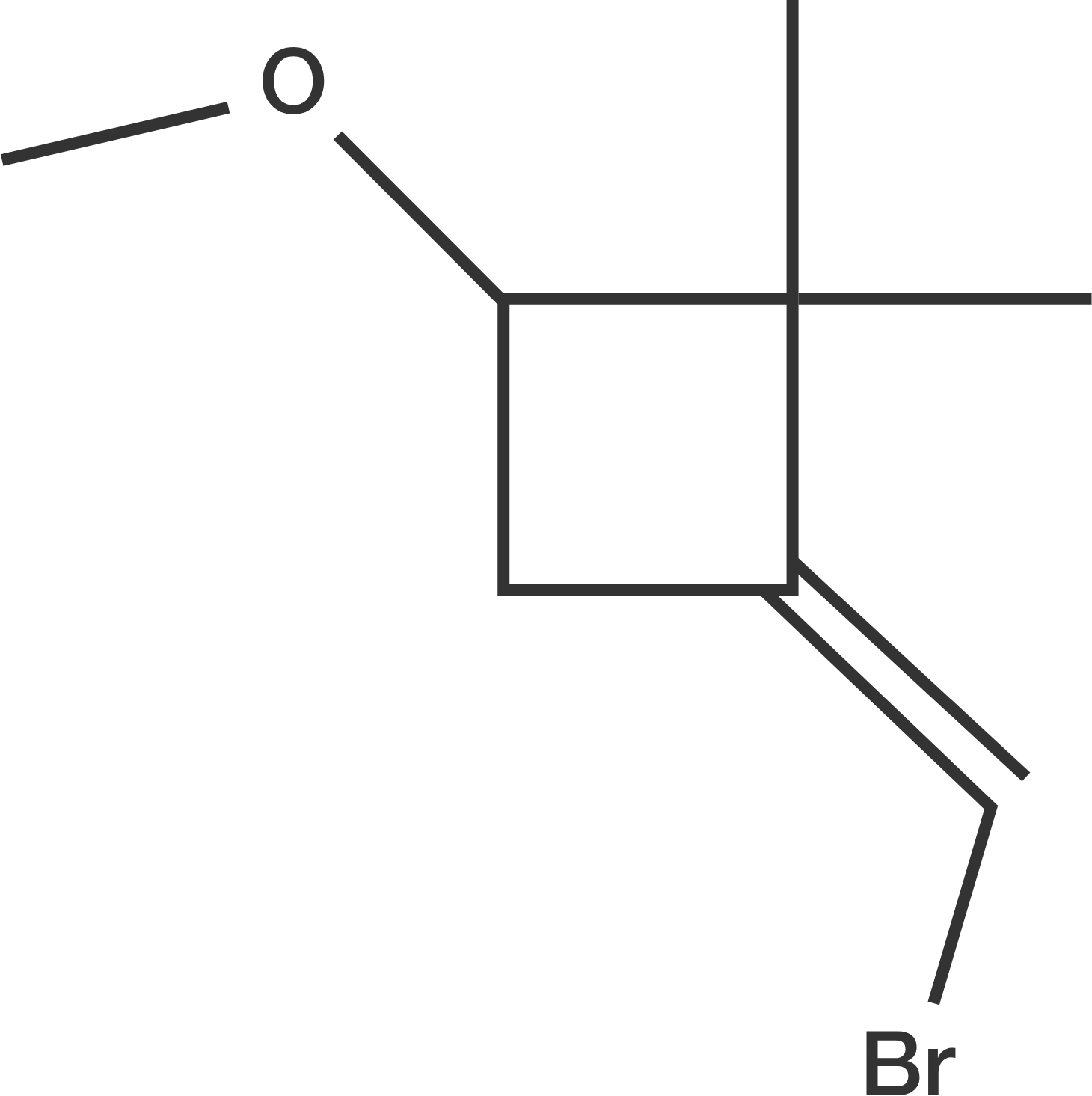# Benzene's Derivative

Chemistry Level 5

Consider the following compound:It undergoes the following reactions with these reagents (in that order):

1. $t$-$\ce{BuOK}$
2. $\ce{CHCl3}$ and $\ce{CH3ONa}$, heat
3. $\ce{H3O+}$ and heat

If the final compound is $x$-chloro $y, z$ dimethyl bromobenzene, what is the value of $x \times y \times z + 16?$

×